# 简介

• 可以增加编程效率。比如可以快速开发拼写纠正程序，而不是通过研究语法来实现逻辑。
• 可以制作更通用的工具。如果已经使用机器学习编写了针对一种语言的拼写纠正程序，那么只要通过提供相应的数据就可以快速实现针对另一种语言的拼写纠正程序。
• 解决无法用常规的逻辑解决的问题。如果没有机器学习，你很难教机器如何识别一个苹果。

# 术语

## 样本（Example）

• 有标签样本：同时包含特征和标签，如一封垃圾邮件或一封正常邮件。
• 无标签样本：仅仅包含特征而没有标签，如一封邮件但我们并不知道是否是垃圾邮件。

## 模型（Model）

• 训练/学习：通过提供大量的样本，让模型学习特征与标签之间的关系。
• 推断：训练完毕后，模型便可以预测无标签样本的标签。

## 回归与分类

• 回归模型（regression model）用来预测连续值：如房价、点击概率。
• 分类模型（classification model）用来预测离散值：如判断邮件是不是垃圾邮件，图片中包含的内容是猫还是狗。

# 线性回归（Linear Regression）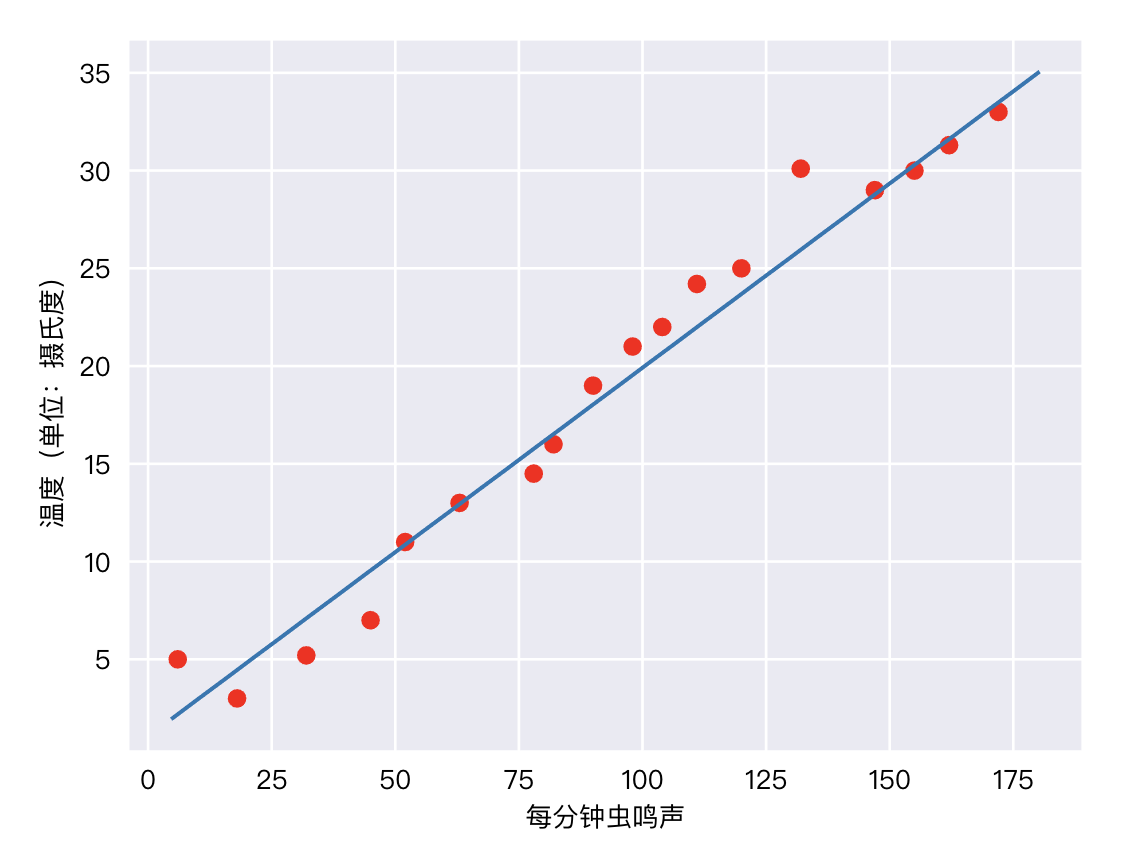$$y = mx + b$$

$$y’ = b + \omega_1x_1$$

• $y’$ 是预测标签。
• $b$ 是偏差（y 轴截距）。
• $\omega_1$ 是特征1 的权重。
• $x_1$ 是特征值。

$$y’ = b + \omega_1x_1 + \omega_2x_2 + \omega_3x_3$$

## 训练（Training）与损失（Loss）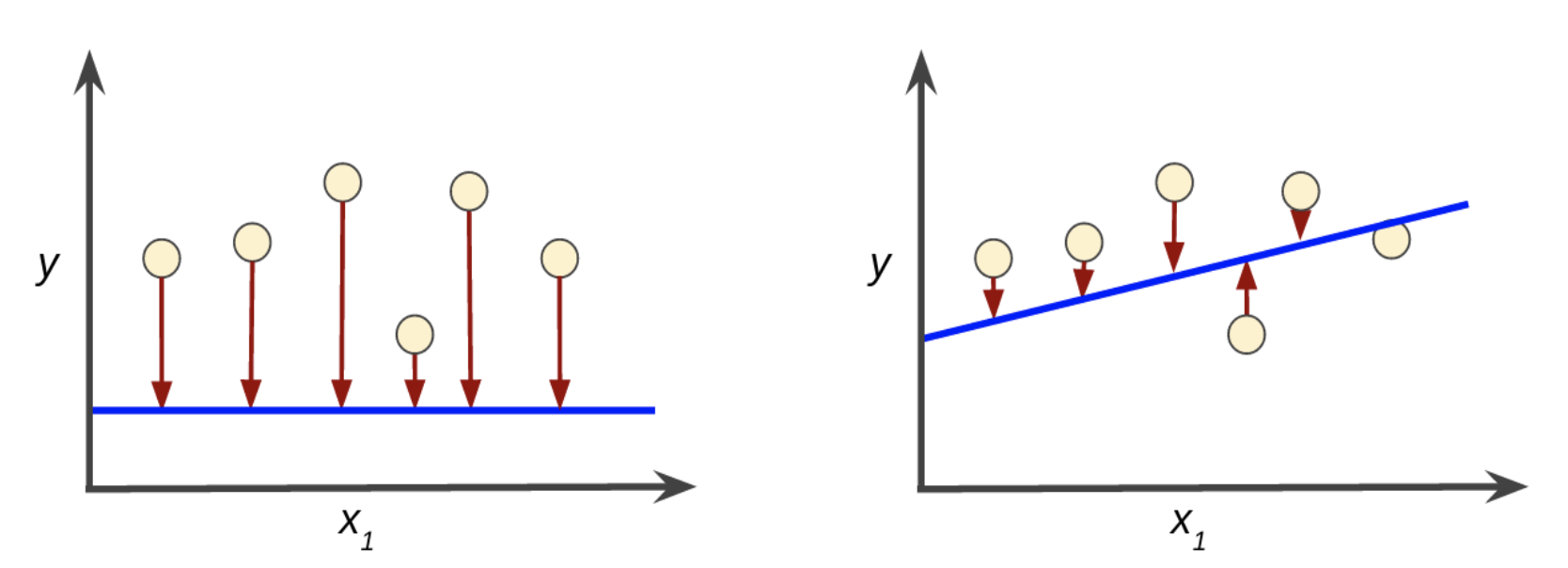$$MSE = \frac{1}{N}\sum_{(x,y) \in D} (y - prediction(x))^2$$

• $(x,y)$ 指的是样本，$x$ 是特征，$y$ 是标签。
• $prediction(x)$ 是模型预测的值。
• $D$ 是样本集。
• $N$ 是样本数量。

# 降低损失

## 迭代方法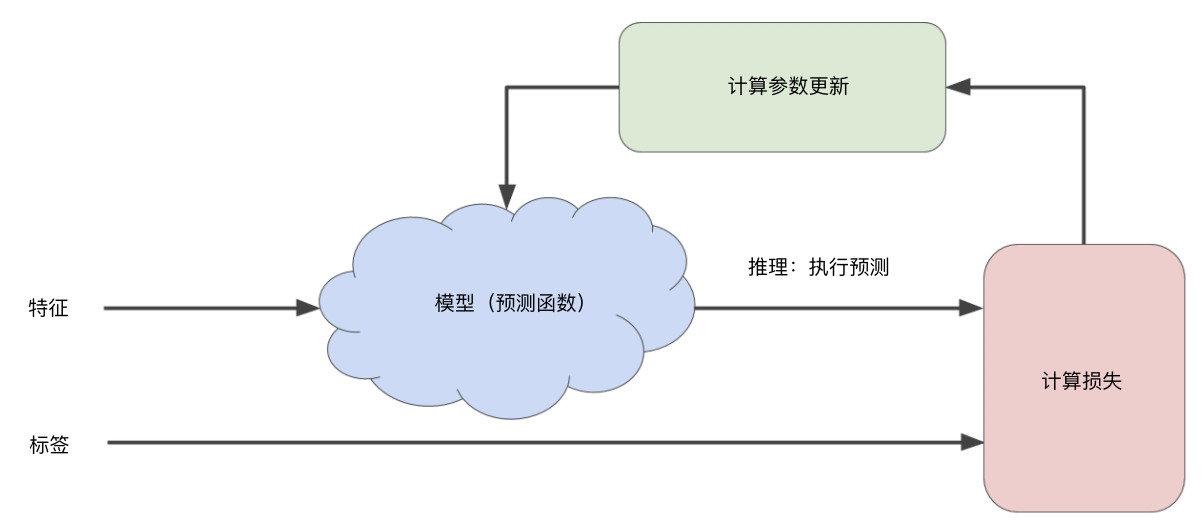$$y’ = b + \omega_1x_1$$

## 梯度下降法（Gradient Descent）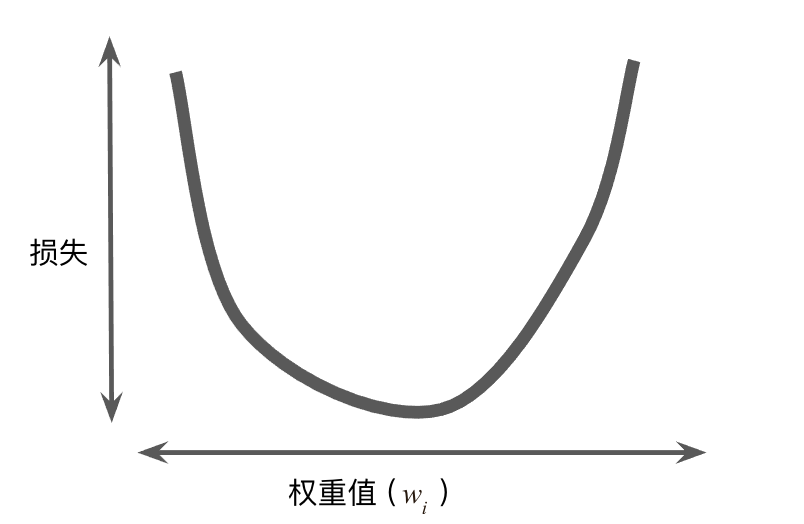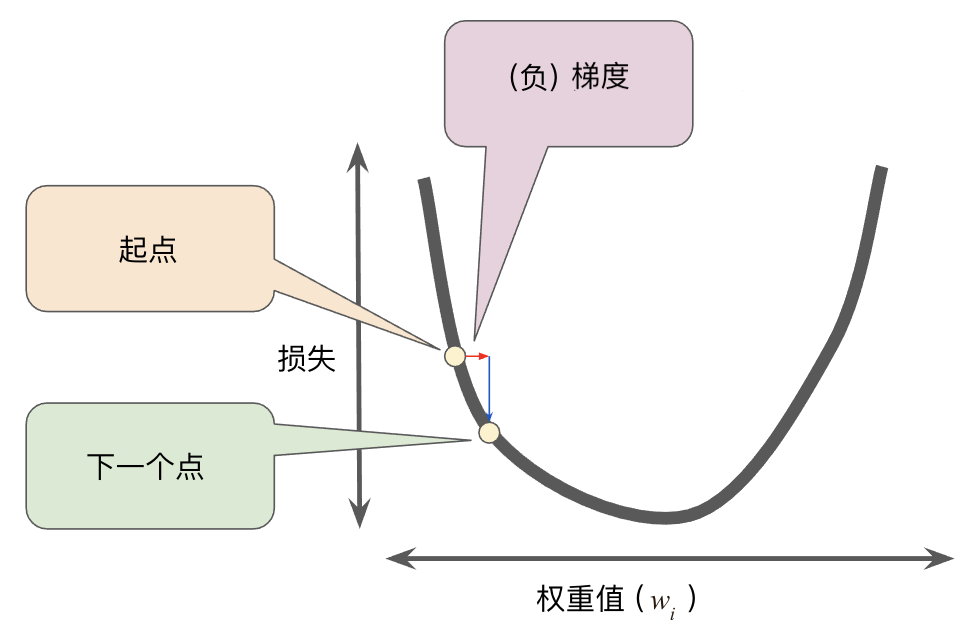## 学习速率（Learning Rate）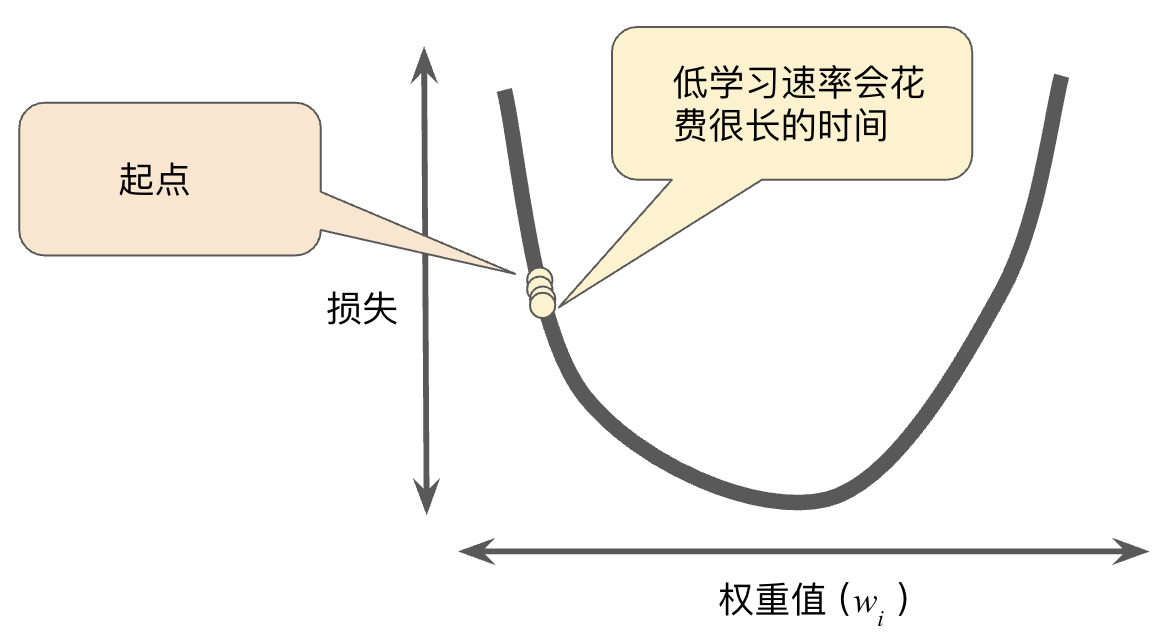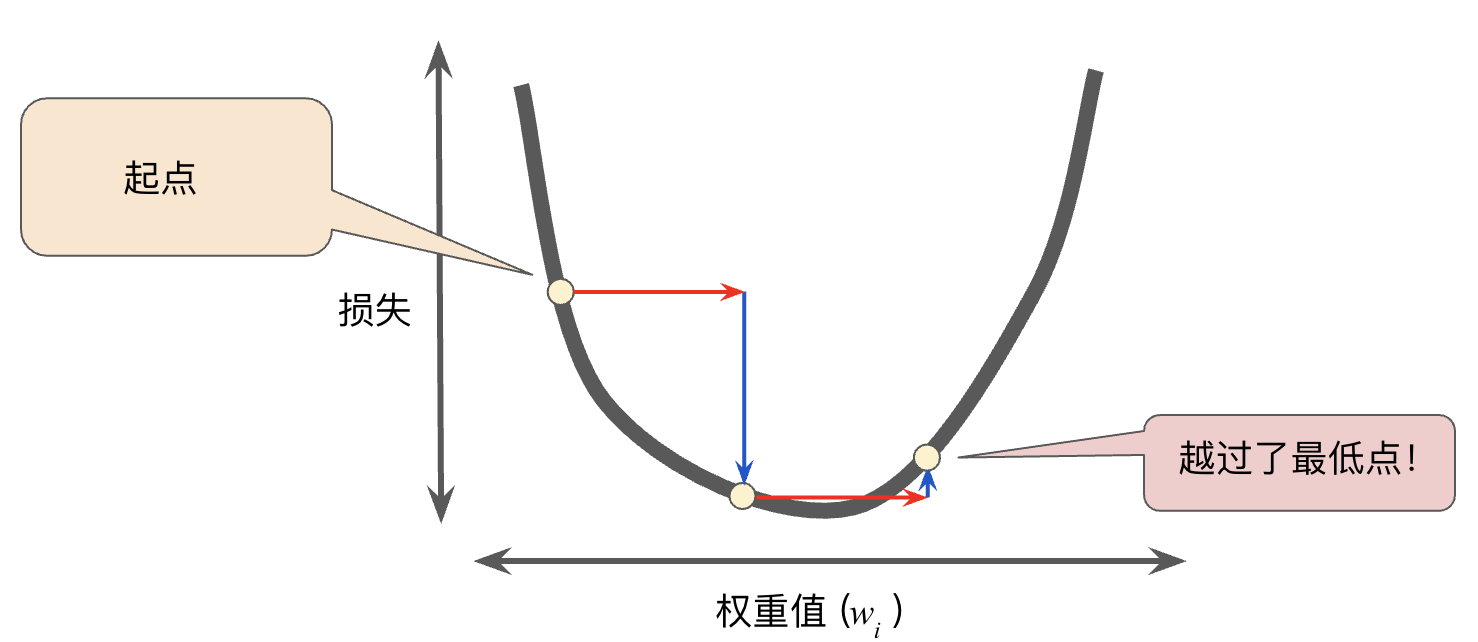## 随机梯度下降法

• 批量梯度下降法（Batch Gradient Descent）：每次迭代计算整个数据集的损失。这样做的好处是可以得到全局最优解，坏处是计算量非常大，并且因为超大批量的数据包含了大量冗余数据，使用起来并不一定比大批量数据好。
• 随机梯度下降法（Stochastic Gradient Descent）：每次迭代只随机抽取一个样本（批量大小为 1）。显然这样做随机性太大，效果也不好。
• 小批量随机梯度下降法（Mini-batch Gradient Descent）：介于前两者之间的方法，每次随机选取 100~1000 个样本。这样兼顾了效率和准确性。